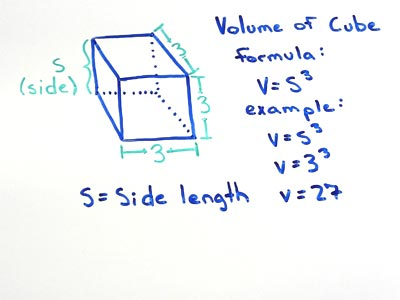# Volume of Cube

## Simple Cube Volume

A cube with a side length (S) of 2 has a volume (V) of 8.

## Cube Volume Formula

for cubes with a volume V and a side length S

• V = S3

substitute the side length S with the measured value, in this example lets use a side length of 4

• V = 43

now cube the side length using the cube function on your calculator or multiplying it by itself three times, this is the cube's volume

• V = 64Given a cube with a side length S the volume can be found by multiplying Side x Side x Side, also written as S3.

### Formulas

What is the formula for the volume of a Cube?

V = S3

What is the formula for the volume of a Rectangular Prism?

V = L x W x H

### Other Formulae

for cubes with side length S

Cube Surface Area

6S2
Cube Face Diagonal
(√2)S
Cube Space Diagonal
(√3)S
S/2
All formulae...